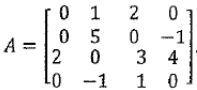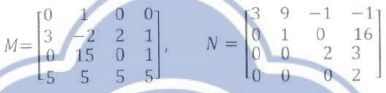Terry Tung>試卷(2022/05/13)

# 主題課程_行列式和線性方程式：行列式#107854

1.

Which of the following statements about the determinant is true?

(A) For any square matrix A, det= -det A.

(B) The determinant of the n x n identity matrix is.

(C) 'The deterrninant of an upper triangular mnatrix is always equal to the product of its
diagonal entries.

(D) Performing a row addition operation on a square matrix does not change its determinant.

(E) Performing a scaling operation on a square matrix does not change its determinant.

2.

Find the determinant for the following matrix(A)-5
(B) 5
(C)1
(D)-1
(E) 0

3.

Givenwhich of the following statements is/are true?
(A) det=det=0.
(B) det= -40.
(C) det= det.
(D) det= 20.
(E) None of the above are true.

4.

Denote det A as the determinant of the matrix A, and denoteas the inverse of the
matrix A. Let A, B, and P be square matrices. Which of the following statements
is/are true?

(A) It is always true that det AB = det BA.

(B) If the columns of A are linearly dependent, then det A = 0.

(C)It is always true that det (A + B) = det A + det B.

(D)If A is invertible, then det(E) Suppose that Pis invertible. Then det= det A.

5.

If there are two nxn matrices A, B and one scalar r,

(A) det= detimplies A = B.

(B) det(rA+B) = rdet+det.

(C)=detdet.

(D) det= detwhere A and B are row equivalent matrices.

(E)6.

Let A, B ∈, where F = C or R. Which of the following statements are true?

(A) tr(AB)= tr(BA)

(B)tr(AB)=trtr(C)= tr(D)(E)tr(A±B)=tr±tr7.

Let A, B ∈, where F = C or R. Which of the following statements are true?

(A) det (AB) = det(BA)

(B) det(AB) = detdet(C) det(B-1AB)= det(D) det= (det)'

(E)det(A±B)=det+det【非選題】
8.
If there are two nxn matrices A, B and one scalar r,

【題組】
(A) det
(A) = det
(B) implies A = B.

(B) det(rA+B) = rdet
(A)+det
(B).

(C) det(AB)T =det
(A)det
(B).

(D) det
(A) = det
(B) where A and B are row equivalent matrices.

(E) det(AB)-) -det(A')det(B' l).

【非選題】
9.【已刪除】【題組】

【非選題】
10.【已刪除】【題組】

【非選題】
11.【已刪除】【題組】

【非選題】
12.

LetCompute the following determinants:

【題組】

(a) det.

【非選題】
13.【題組】

(b) det.

【非選題】
14.【題組】

(c) det.

【非選題】
15.【題組】

(d)【非選題】
16.

Let A, B, C, and D be nxn matrices with A invertible. Prove that det(det A) det() .

【非選題】
17.

Given a n X n tridiagonal matrix A as below:Please find the determinant of A when n = 2020.

【非選題】
18.
Prove or disprove the following statements:

【題組】(a) There exist nxn real matrices A and B such that AB-BA=I, where I is then nxn identity matrix.

【非選題】
19.【題組】(b) If A and Bare nxn matrices and det(A-B)=0,then det
(A)=det
(B).

【非選題】
20.【題組】

(c) If A is a nilpotent matrix (namely, A is an nxn matrix such that= O for some positive integer k), then det(I + A)=1 , where I is the nxn identity matrix.

【非選題】
21.

Find the values of x such that the given matrix is not invertible.【非選題】
22.

Compute the determinant of【非選題】
23.

Let B be the n x n matrix, compute det
(B).【非選題】
24.

Given two matrices M and N, please calculate【題組】(a)  det(M)

【非選題】
25.【題組】(b)det(MN)

【非選題】

【非選題】
27.
4.Let A and B be 3 x3 matrices with det
(A) = 5, det
(B) = 10, and det(A+B) = 60. Decide the following values.

【題組】(a) det(A+A).

【非選題】
28.【題組】(b)  det(A2B+AB2).

【非選題】
29.【題組】

Iffind det(K).

【非選題】
30.

Letand a,b ∈. Find det
(A).

【非選題】
31.

Solve for t if det=0

【非選題】
32.

Letandbe the nxn identity matrix. Find det(A +.).

【非選題】
33. Please find the determinant of A.【非選題】
34.

Prove that det(M)=for the following matrix:【非選題】
35.

Suppose that Px(xt) is a polynomial of order & with leading coefficients, ax, k = 0,ㆍㆍ.,n -1.
That is,+...+ a1x+ a0, k = 0,.n -1. Then=_______

### 懸賞詳解

#### 國一自然下第二次

18. (甲)始新馬；(乙)中新馬；(丙)鮮新馬；(丁)現代馬；(戊)漸新馬，馬的出 現年代順序排列為何？ (A)甲乙丙丁戊 (B)甲丁戊丙乙 (C)甲戊乙丙丁 (D)丁丙�...